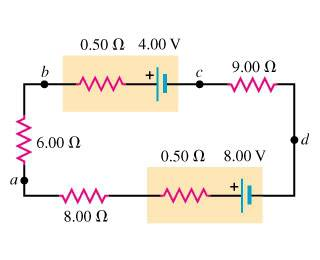# Terminal Voltage and Current

Soaring Crane

## Homework Statement

a. What is the potential difference V_ad in the circuit of the figure?b. What is the terminal voltage of the 4.00 - V battery?

c. A battery with emf 10.3 V and internal resistance 0.50 ohm is inserted in the circuit at d, with its negative terminal connected to the negative terminal of the 8.00 - V battery. What is the difference of potential V_bc between the terminals of the 4.00 - V battery now?

See below.

## The Attempt at a Solution

I really need help with my signs. Please direct me if I am incorrect on the different parts.

a. Setting I as clockwise,

-0.50 ohm*(I) – 4.00 V – 9.00 ohm*(I) + 8.00 V – 0.5 ohm*(I) – 8.00 ohm*(I) – 6.00 ohm*(I) = 0

I = (-4.00 V)/(-24 ohm) = 0.16667 A ???

Using this,

Vad = -8 V + (8.50 ohm)*I = -8 V + (1.417 V) = -6.583 V???

b. V_bc for battery = -E + I*r = -4.00 V + (0.50 ohm)*(0.16667 A) = -3.917 V???

c. The new I must be found? Therefore,

-0.50 ohm*(I) – 4.00 V - 9.00 ohm*(I) - 0.50 ohm*(I) – 10.3 V + 8.00 V - 8.00 ohm*(I) - I(6.00 ohm) - 0.50 ohm*(I) = 0

I*(-24.5 ohm) = 6.3 V

I = 6.3 V/-24.5 ohm = -0.2571 A ??? Thus, the current actually flows counterclockwise?

V_bc = -E + I*r = -4.00 V + (0.50 ohm)*(0.2571A) = -3.8714 V???

Thank you.

Last edited:

Mindscrape
Signs don't really matter as long as you are consistent. Here is the way I like to remember it:

When you are doing voltage loops, are you measuring the voltage drop or voltage gain? Voltage is the potential difference, so you want the difference to be postive. So if you guess your current will go clockwise, then all the resistor elements the current goes through will have a positive drop, and any voltage gains will be negative.

You did the opposite, which will work as long as you remember your method, which could be just as valid. However, it doesn't look like you remained consistent. You found the current going 'clockwise' in the first part by making voltage drops negative, and gains positive. Then solving for Vad it looks like you reversed it, and made your gains negative and drops positive. Right idea though.

b) Yes, you did the relative potential correct, but I personally think your way is sort of wacky (maybe just because I am used to mine). Just remember that in your way you have to change it back in the end.

c) I think you did it right, the answer makes sense since the larger voltages are going in the counter clockwise direction.

I would just stick with convention if I were you. So, current going across a resistor will have a positive voltage, and voltage gains will really have a negative sign as you go around the loop. Even if your way makes sense now, if you go against convention for so long then you will forget which parts are actually backwards and which aren't when you look at examples.

Soaring Crane
Since the current is moving in the clockwise direction for the first two parts, this is why the terminal voltage of the 4.00-V battery is negative (moving from the positive terminal to the negative terminal)?

Thanks again.

Mindscrape
So for your convention you found the potential between b and c, but because your convention was backwards your answer was backwards. Let me put this into words. You showed that there was a 3.917 voltage drop because you made all the voltage drops negative; however, the convention is to make the potential difference positive.